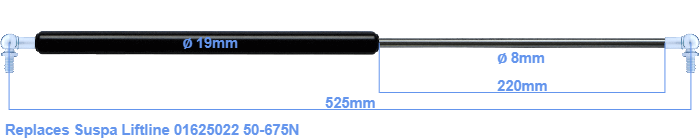# Replacement for Suspa Liftline 01625022 50-675N

\$53.02

Replacement gas spring for the Suspa Liftline 01625022 50-675 Newton. This fits a (possibly already present) ball with a diameter of 10mm. A corresponding ball is included. Brand: Hahn.
 Force Choose an option50 Newton60 Newton80 Newton100 Newton120 Newton140 Newton150 Newton160 Newton180 Newton200 Newton220 Newton240 Newton250 Newton260 Newton280 Newton300 Newton320 Newton340 Newton350 Newton360 Newton380 Newton400 Newton420 Newton440 Newton450 Newton460 Newton480 Newton500 Newton520 Newton540 Newton550 Newton560 Newton580 Newton600 Newton620 Newton640 Newton650 Newton660 Newton675 NewtonClear
This gas spring is also known as 16-2 016 25022, 16-2-264-225-A246-B246.The diameter of the cylinder of this gas spring is 19 mm. The rod has a diameter of 8 mm. The rod (the chrome part) is 220 mm long. The total length of this replacement is exactly 525 mm. Attention: this is the length between the centers of the mounting parts. Without the mounting parts this gas spring is 485 mm long (thread to thread). The force of this replacement gas spring is 50-675 Newton. This gas spring can replace a Suspa Liftline gas spring, but is not an orignal Suspa Liftline one. This replacement gas spring is a Stabilus Industry Line gas spring. The gas spring can be used without problems as a replacement, because the dimensions and force equal.
Category: